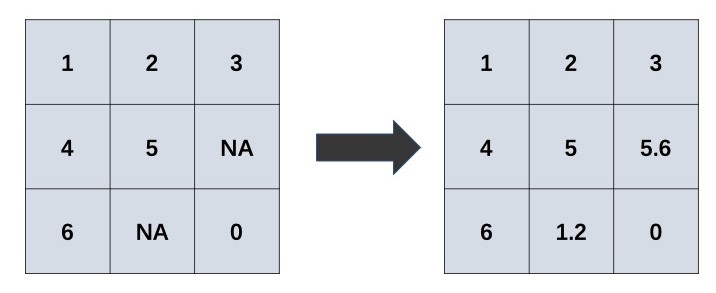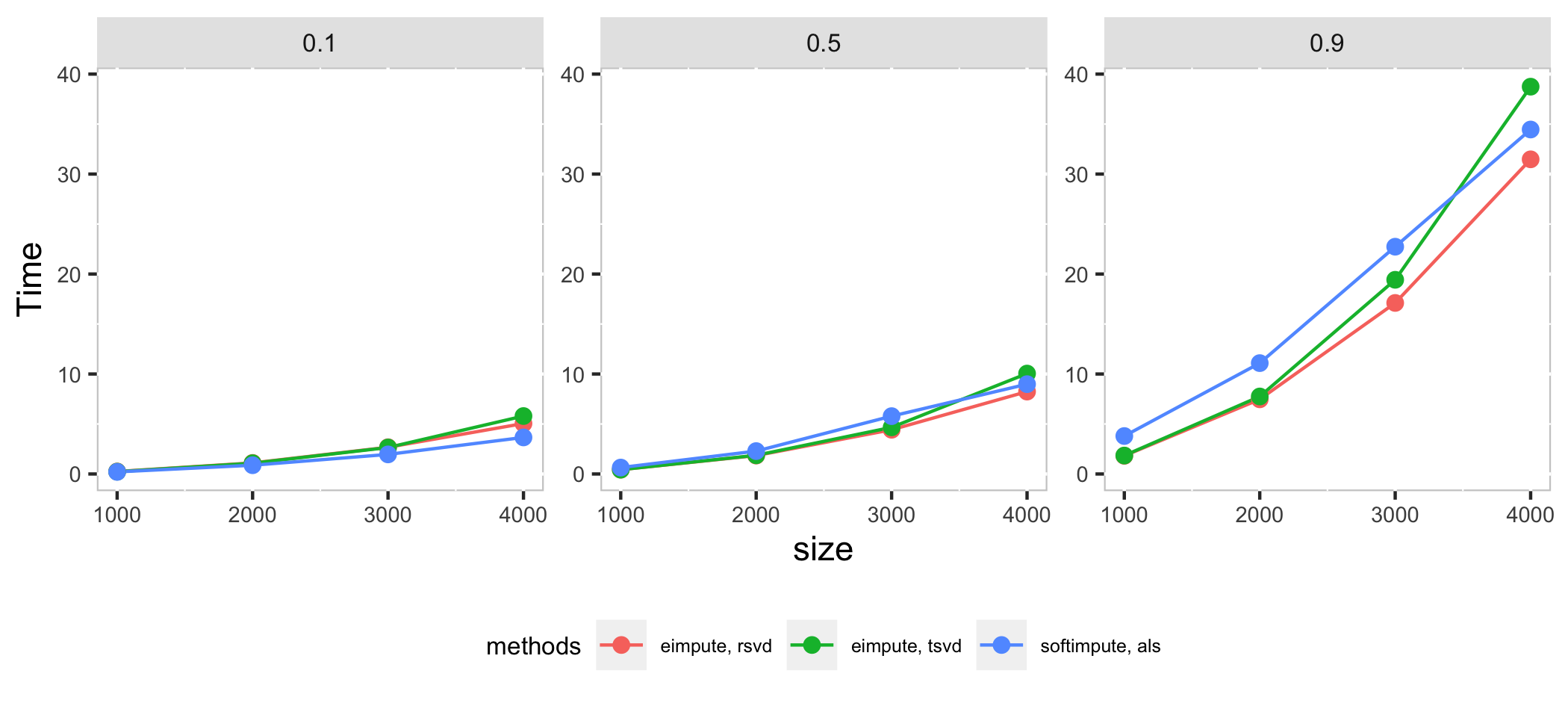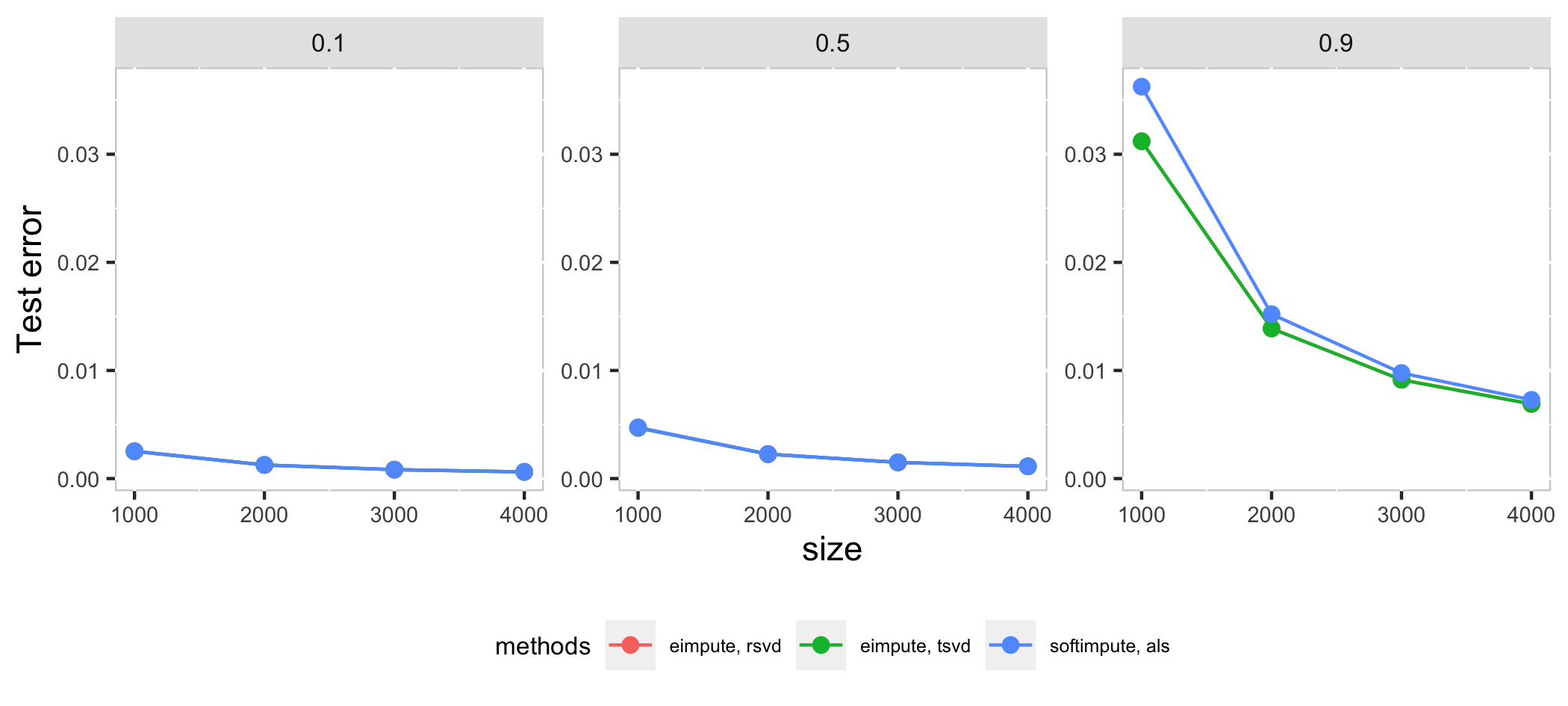# eimpute: Efficiently IMPUTE Large Scale Incomplete Matrix

## Introduction

Matrix completion is a procedure for imputing the missing elements in matrices by using the information of observed elements. This procedure can be visualized as:Matrix completion has attracted a lot of attention, it is widely applied in:

• tabular data imputation: recover the missing elements in data table;
• recommend system: estimate users’ potantial preference for items pending purchased;
• image inpainting: inpaint the missing elements in digit images.

A computationally efficient R package, eimpute is developed for matrix completion. In eimpute, matrix completion problem is solved by iteratively performing low-rank approximation and data calibration, which enjoy two admirable advantages:

• unbiased low-rank approximation for incomplete matrix
• less time consumption via truncated SVD

Compare eimpute and softimpute in systhesis datasets $$X_{m \times m}$$ with $$p$$ proportion missing observations. The square matrix $$X_{m \times m}$$ is generated by $$X = UV + \epsilon$$, where $$U$$ and $$V$$ are $$m \times r$$, $$r \times n$$ matrices whose entries are $$i.i.d.$$ sampled standard normal distribution, $$\epsilon \sim N(0, r/3)$$.

• $$m$$ is chosen as 1000, 2000, 3000, 4000
• $$p$$ is chosen as 0.1, 0.5, 0.9.In high dimension case, als method in softimpute is a little faster than eimpute in low proportion of missing observations, as the proportion of missing observations increase, rsvd method in eimpute have a better performance than softimpute in time cost and test error. Compare with two method in **eimpute*, rsvd method is better than tsvd in time cost.

## Installation

Install the stable version from CRAN:

install.packages("eimpute")

Install the development version from github:

library(devtools)
install_github("Mamba413/eimpute", build_vignettes = TRUE)

## Quick Example

We start with a toy example. Let us generate a small matrix with some values missing via incomplete.generator function.

m <- 6
n <- 5
r <- 3
x_na <- incomplete.generator(m, n, r)
x_na
#>            [,1]       [,2]       [,3]      [,4]       [,5]
#> [1,] -0.8269428  1.2228586         NA        NA         NA
#> [2,] -2.2410010  4.5095165         NA        NA         NA
#> [3,]  0.4499102         NA -0.2818085 0.7718102 -0.8364048
#> [4,]         NA  1.7167365  0.9480745        NA  3.5680208
#> [5,]         NA  0.7240437         NA        NA  0.2633712
#> [6,]         NA -2.8879249         NA 1.2027552         NA

Use eimpute function to impute missing values.

x_impute <- eimpute(x_na, r)
x_impute[["x.imp"]]
#>            [,1]       [,2]        [,3]      [,4]       [,5]
#> [1,] -0.8269428  1.2228586  0.19035820 0.9514541  0.2994880
#> [2,] -2.2410010  4.5095165  0.39560039 0.7295574  0.4911418
#> [3,]  0.4499102 -1.2083884 -0.28180850 0.7718102 -0.8364048
#> [4,] -0.3408353  1.7167365  0.94807452 0.1835412  3.5680208
#> [5,] -0.3669454  0.7240437  0.11988844 0.3294654  0.2633712
#> [6,]  1.3875965 -2.8879249  0.01871091 1.2027552  0.4512052